# How to Find Length of String in Bash Script?

• Last Updated : 30 Jan, 2022

In this article, we are going to see how to get string length in Bash scripting.

Here are a few ways from which we can get the length of the string in BASH:

1. Using the # operator
2. Using while loop
3. Using expr command
4. Using awk command
5. Using wc command

Using these mentioned tools and commands, we will be able to get the length of a string variable in the BASH script.

## Method 1: Using the # operator

```#!usr/bin/env bash

str="this is a string"
n=\${#str}
echo "Length of the string is : \$n "```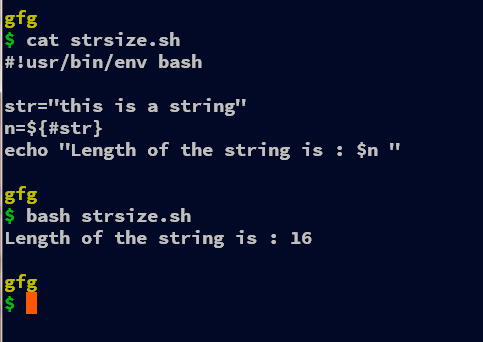We can use the # operator to get the length of the string in BASH, we need to enclose the variable name enclosed in “{ }” and inside of that, we use the # to get the length of the string variable. Thus, using the “#” operator in BASH, we can get the length of the string variable.

## Method 2: Using while loop

In this example. we can use the while loop along with the read command to obtain the length of the string. Firstly we initialize the string to whatever you like, it can be a user input, positional parameter, etc. It’s quite easy to work with the variable, so therefore we create a variable for the string and also initialize the length “n” to 0. Then we start the while loop by reading characters by character using the argument -n1 i.e read just the single character from the entire string.

For every character in the variable “str” i.e. after every iteration, we increment the length “n” by one. In the while loop, we are reading from the string “str” with echo command along with -n argument which restricts to escape the newline character. Thus at the end of the loop, we have the length of the string in the variable n and can be accessed as per requirements.

```#!usr/bin/env bash

str="this is a string"
n=0
n=\$((n+1));
done < <(echo -n "\$str")
echo "Length of the string is : \$n "```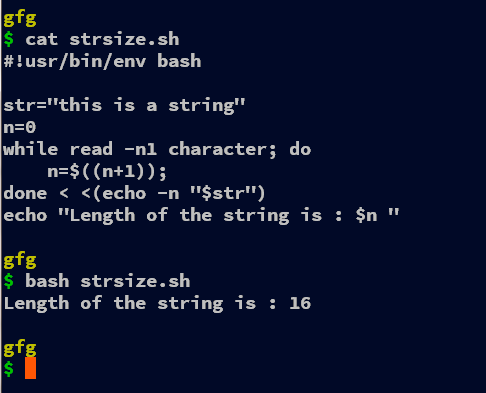## Method 3: Using expr command

```#!usr/bin/env bash

str="Test String@#\$"
n=`expr "\$str" : '.*'`
echo "Length of the string is : \$n "```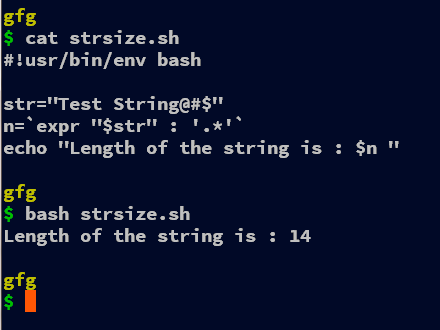In this example, we are using the expr command to find the length of the string in BASH. The expr command takes the first argument as the string variable and then the comparison operator “:” that counts the number of common characters in the string and the next string provided after the “:” operator.  For calculating the length of the string, we parse the previous string. For that “.*” is the regex that gets 0 or more characters of the previously provided token. Even parsing the string instead of “.*” will work

`n=`expr "\$str" : "\$str"``

Here we have used the backticks(“` `“) but even using the \$( ) there is no such advantage of using the former over the latter. Both serve the same purpose but the \$( ) nests very effective than the backticks.

```#!usr/bin/env bash

str="Test String@#\$"
n=`expr length "\$str"`
echo "Length of the string is : \$n "```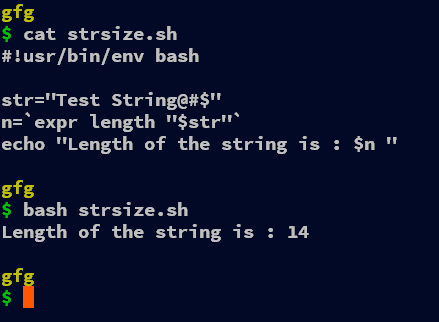We can even use the built-in function in expr command “length” that gets the length of the string as a parameter just after the function. The length command as the name suggests gives us the length of the provided string or any variable.

Remember for every code snippet following this one, you can use the \$( ) instead of backticks(` `).

`n=\$(expr length "\$str")`

## Method 4: Using awk command

```#!usr/bin/env bash

str="this is a string"
n=`echo \$str | awk '{print length}'`
echo "Length of the string is : \$n "```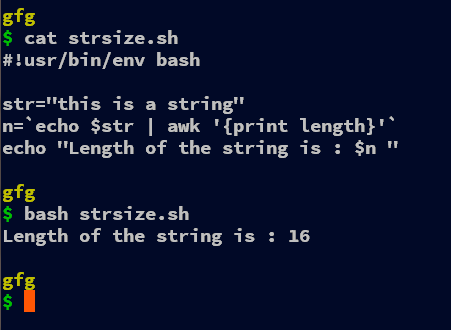We can even use the awk command to get the length of the string. We use the function length which is built-in awk along with print that prints the value and thus stores the length of the string after piping (“|“) with the echo command.

In the expression, echo \$str | awk ‘{print length}’ we echo the string variable “str” and then redirect (pipe) the output of it to the awk command. In the awk command, we print the output of the length function, which takes the str variable and stores it in the variable “n“.

## Method 5: Using wc command

```#!usr/bin/env bash

str="this is a string"
n=`echo -n "\$str"|wc -c`
echo "Length of the string is : \$n "```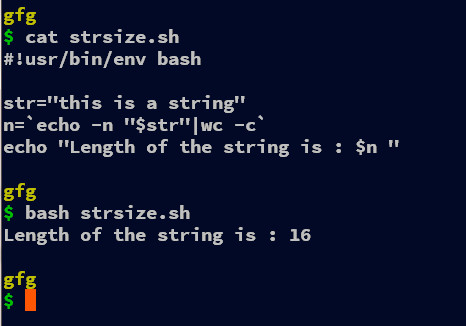In this demonstration, we have used the wc command to get the length of the string. Inside of this command, “echo -n “\$str” | wc -c” the echo command is given an argument -n which doesn’t escape the new line character. Next, the output of the echo command is redirected (piped) to the wc command which again is given the argument -c which counts the number of bytes in the provided variable, we can even use -m which also precisely counts the number of characters.

`echo -n "\$str" | wc -m`
My Personal Notes arrow_drop_up